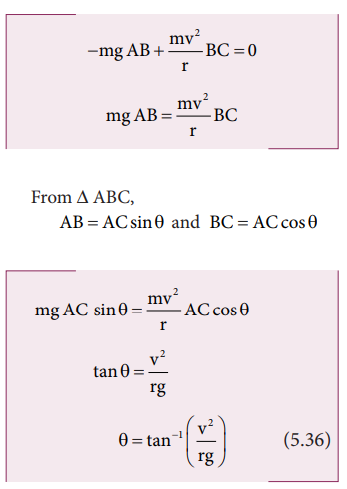Home | | Physics 11th std | Bending of Cyclist in Curves

# Bending of Cyclist in Curves

Let us consider a cyclist negotiating a circular level road (not banked) of radius r with a speed v.

Bending of Cyclist in Curves

Let us consider a cyclist negotiating a circular level road (not banked) of radius r with a speed v. The cycle and the cyclist are considered as one system with mass m. The center gravity of the system is C and it goes in a circle of radius r with center at O. Let us choose the line OC as X-axis and the vertical line through O as Z-axis as shown in Figure 5.19.The system as a frame is rotating about Z-axis. The system is at rest in this rotating frame. To solve problems in rotating frame of reference, we have to apply a centrifugal force (pseudo force) on the system which will be mv2/r

This force will act through the center of gravity. The forces acting on the system are, (i) gravitational force (mg), (ii) normal force (N), (iii) frictional force (f) and (iv) centrifugal force (mv2/r) . As the system is in equilibrium in the rotational frame of reference, the net external force and net external torque must be zero. Let us consider all torques about the point A in Figure 5.20.For rotational equilibrium,The torque due to the gravitational force about point A is ( mgAB) which causes a clockwise turn that is taken as negative. The torque due to the centripetal force is ( mv2/r BC) which causes an anticlockwise turn that is taken as positive.While negotiating a circular level road of radius r at velocity v, a cyclist has to bend by an angle θ from vertical given by the above expression to stay in equilibrium (i.e. to avoid a fall).

## Solved Example Problems for Bending of Cyclist in Curves

### Example 5.13

A cyclist while negotiating a circular path with speed 20 m s-1 is found to bend an angle by 30o with vertical. What is the radius of the circular path? (given, g = 10 m s-2)

### Solution

Speed of the cyclist, v = 20 m s-1

Angle of bending with vertical, θ = 30oTags : Physics , 11th Physics : UNIT 5 : Motion of System of Particles and Rigid Bodies
Study Material, Lecturing Notes, Assignment, Reference, Wiki description explanation, brief detail
11th Physics : UNIT 5 : Motion of System of Particles and Rigid Bodies : Bending of Cyclist in Curves | Physics